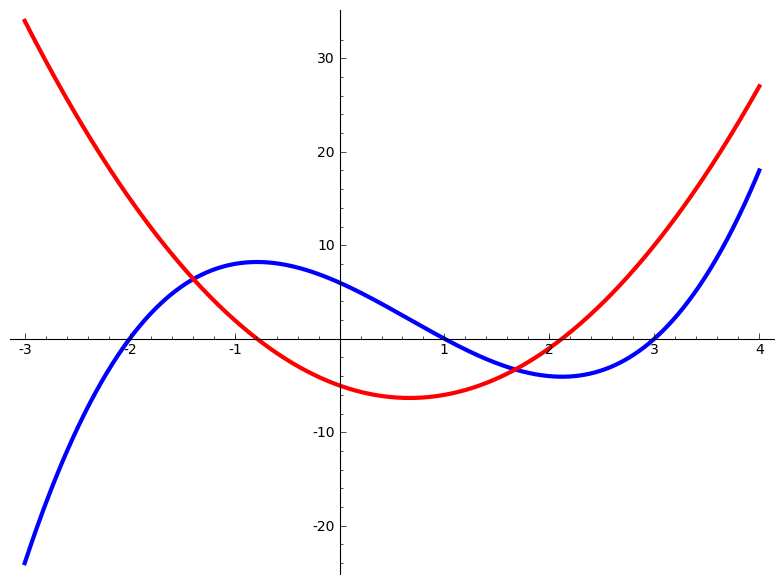View Source

### Subsection11.1Specialized Subdivisions

In a longer work you might wish to have some references on a per-chapter basis, or similar. You can make a “references” subdivision anywhere to hold bibliographic items, and you can reference the items like any other item. For example, we can cite the article below [11.4.3, Chapter R], included an indication that a specific chapter may be relevant.

### Exercises11.2Exercises

#### 1.

No problem here, but the next two are in an “exercise group” with an introduction and a conclusion, along with an optional title. The two problems of the exercise group should be indented some to indicate the grouping.

N.B. An <exercisegroup> is meant to hold a collection of (short) exercises with common, shared, instructions. Do not use this structure to subdivide an <exercises> division, as you will eventually be disappointed. Instead, use the available, but under development as of 2019-11-02, <subexercises>, which requires a <title>.

#### Two Derivative Problems.

In the next two problems compute the indicated derivative.You could “connect” the image above with the exercises following as part of this introduction for the exercisegroup.

##### 2.

$$f(x)=x^3\text{,}$$ $$\frac{df}{dx}\text{.}$$ This sentence is just a bunch of gibberish to check where the second line of the problem begins relative to the first line.

We cross-reference the next problem in this exercise group. For the phrase-global form, the common element of the cross-reference and the target should be the exercises division, and not the enclosing exercisegroup: Exercise 3 of Exercises 11.2.

##### 3.

$$y = \cos(x)\text{,}$$ $$y^\prime\text{.}$$

Note that the previous two problems used very different notation for the function and the resulting derivative.

#### 4.

This isn't really an exercise, but an explanation that the next <exercisegroup> has a title and no <introduction>, which once resulted in some aberrant formatting in output.

#### Two More Derivative Problems.

Some common instructions would go here in the <introduction>

##### 5.

$$f(x)=x^3\text{,}$$ $$\frac{df}{dx}\text{.}$$ This sentence is just a bunch of gibberish to check where the second line of the problem begins relative to the first line.

We cross-reference the next problem in this exercise group. For the phrase-global form, the common element of the cross-reference and the target should be the exercises division, and not the enclosing exercisegroup: Exercise 3 of Exercises 11.2.

##### 6.

$$y = \cos(x)\text{,}$$ $$y^\prime\text{.}$$

#### 7.

Compute $$\int 3x^2\,dx\text{.}$$

#### 8.

One of the few things you can place inside of mathematics is a “fill-in” blank. We demonstrate a few scenarios here. See details on syntax in Subsection 4.7–the use is identical within mathematics.

• Inside inline math (short, space for $$x$$): $$\sin(\fillinmath{x})$$

• Inside inline math (default, space for $$XXX$$): $$\sin(\fillinmath{XXX})$$

• Inside exponents and subscripts (each is space for the string “12”). In this case, be sure to wrap your exponents and subscripts in braces, as would be good practice anyway: $$x^{5+\fillinmath{12}}\,y_{\fillinmath{12}}$$

• Inside inline math (too long for this line probably, 40 characters long): $$\tan(\fillinmath{Mxxxxxxxxxxxxxxxxxxxxxxxxxxxxxxxxxxxxxxx})$$

• So use inside a displayed equation

\begin{equation*} 16\log\space\fillinmath{1-x^2} \end{equation*}

like this one.

• Inside the second line of a multi-line display:

\begin{align*} y &= x^7\,x^8\\ &= x^{\fillinmath{15}} \end{align*}
• This fillin has the historical @characters attribute for a fillin inside math: $$1+\fillinmath{XXXXX}+4=10\text{,}$$ which may be more convenient, but may not side properly in places like subscripts, superscripts, fractions, limits of integrals, and so on.

### Exercises11.3More Exercises

#### 1.

This is not a real exercise, we just want to explain that this is another subsection of exercises, which has two consecutive exercise groups.

#### Exercise Group.

Introduction to first exercise group.

##### 2.

Only exercise of first group.

Conclusion to first exercise group.

#### Exercise Group.

Introduction to second exercise group.

##### 3.

First exercise of second group.

##### 4.

Second exercise of second group.

Conclusion to second exercise group.

#### Exercise Group.

An <exercisegroup> can have a cols attribute taking a value from 2–6. Exercises will progress by row, in so many columns. On a small screen, the HTML exercises may reorganize into fewer columns.

This feature was designed with short “drill” exercises in mind. With long exercises, or exercises with long hints, answers, or solutions, there is a risk that the output will have bad page breaks in the vicinity (just before) such an exercise that occupies too much vertical space. Edit, rearrange, or use fewer columns to see if the situation improves.

#### 25.

Make a table and a graph for the function $$f(x)=x^2\text{.}$$

$$x$$ $$f(x)$$
$$0$$ $$0$$
$$1$$ $$1$$
$$2$$ $$4$$
$$3$$ $$9$$### References11.4References

These items are here to test basic formatting of references.



Gilbert Strang, The Fundamental Theorem of Linear Algebra, The American Mathematical Monthly November 1993, 100 no. 9, 848–855.


J. B. Conrey and D. W. Farmer, Mean values of $$L$$-functions and symmetry, Internat. Math. Res. Notices no. 17, (2000) pp. 883-908.


Robert A. Beezer, A First Course in Linear Algebra, 3rd Edition, Congruent Press, 2012.
Note.

An online, open-source offering.



H. Davenport, Multiplicative Number Theory, GTM 74 Springer-Verlag New York, NY; (2000) xiv+177. [A note may accompany a bibliographic item, such as saying the manuscript is under review. But it cannot contain any formatting.]


Alexander Rosswell, Diffeomorphisms of Penciled Fiber Bundles, Mathematicians of America (2020), 2 no. 6, 884–888.


Ibid., Diffeomorphisms of Penciled Fiber Bundles, Part 2, Mathematicians of America (2021), 3 no. 4, 102–103.

This is a conclusion, which has not been used very much in this sample. Did you see that the entry for [11.4.3] has a short annotation? So you can make annotated bibliographies easily.